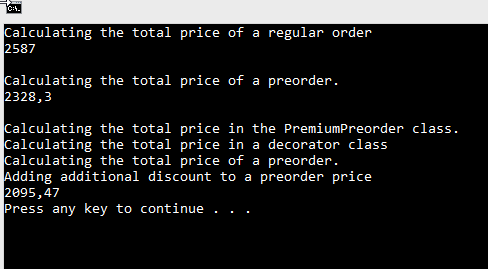## About the Decorator Design Pattern

A Decorator is a structural design pattern that allows us to extend the behavior of objects by placing these objects into a special wrapper class.

The Decorator design pattern is quite popular in C# due to the fact that it helps us dynamically add behaviors to the wrapped objects.

The structure of this pattern consists of a Component class and a Concrete Component class from one part and a Decorator class and a Concrete Decorator class on the other side. The Concrete Decorator class is going to add additional behavior to our Concrete Component.

So, when do we use this pattern?

Well, we should use this pattern when we have a need to add additional behavior to our objects. Furthermore, we should use it when it is too complicated to use inheritance or when it doesn’t make sense at all (too many inherit layers, urge to modify existing inheritance hierarchy by adding some additional layers, etc.).

We are going to see how all these elements work together inside the Decorator design pattern through the practical example, which is going to make this pattern easier to comprehend.

## Decorator Design Pattern Implementation

Let’s imagine that we have a simple application to calculate the total order price in our shop. But also, we have some additional requests. If a buyer orders our products in a preorder period we are going to give a 10 percent discount. So, let’s start first with our Component class:

Component class
 1 2 3 4 5 6 7 8 9 10 11 `public` `abstract` `class` `OrderBase` `{` `    ``protected` `List products = ``new` `List` `    ``{` `        ``new` `Product {Name = ``"Phone"``, Price = 587},` `        ``new` `Product {Name = ``"Tablet"``, Price = 800},` `        ``new` `Product {Name = ``"PC"``, Price = 1200}` `    ``};` ` ` `    ``public` `abstract` `double` `CalculateTotalOrderPrice();` `}`

The Component class contains functionality that will be shared with other Concrete Component classes. Having that in mind, let’s create the Concrete Components:

RegularOrder class
 1 2 3 4 5 6 7 8 `public` `class` `RegularOrder : OrderBase` `{` `    ``public` `override` `double` `CalculateTotalOrderPrice()` `    ``{` `        ``Console.WriteLine(``"Calculating the total price of a regular order"``);` `        ``return` `products.Sum(x => x.Price);` `    ``}` `}`
Preorder class
 1 2 3 4 5 6 7 8 `public` `class` `Preorder : OrderBase` `{` `    ``public` `override` `double` `CalculateTotalOrderPrice()` `    ``{` `        ``Console.WriteLine(``"Calculating the total price of a preorder."``);` `        ``return` `products.Sum(x => x.Price) * 0.9;` `    ``}` `}`

This code is pretty clean and easy to understand. We just override our abstract method `CalculateOrderPrice` and calculate the total price. So, right now, we can start using these concrete components:

Implementation
 1 2 3 4 5 6 7 8 9 10 11 12 13 `class` `Program` `{` `    ``static` `void` `Main(``string``[] args)` `    ``{` `        ``var regularOrder = ``new` `RegularOrder();` `        ``Console.WriteLine(regularOrder.CalculateTotalOrderPrice());` `        ``Console.WriteLine();` ` ` `        ``var preOrder = ``new` `PreOrder();` `        ``Console.WriteLine(preOrder.CalculateTotalOrderPrice());` `        ``Console.WriteLine();` `    ``}` `}`

This works just fine.

But now, we receive an additional request to allow an additional 10 percent discount to our premium users for the preorder. Of course, we could only change the `Preorder` class with one if statement to check if our user is a premium user, but that would break the Open Closed Principle. So, in order to implement this additional request, we are going to start with a Decorator class which is going to wrap our `OrderBase` object:

OrderDecorator class
 1 2 3 4 5 6 7 8 9 10 11 12 13 14 15 `public` `class` `OrderDecorator : OrderBase` `{` `    ``protected` `OrderBase order;` ` ` `    ``public` `OrderDecorator(OrderBase order)` `    ``{` `        ``this``.order = order;` `    ``}` ` ` `    ``public` `override` `double` `CalculateTotalOrderPrice()` `    ``{` `        ``Console.WriteLine(\$``"Calculating the total price in a decorator class"``);` `        ``return` `order.CalculateTotalOrderPrice();` `    ``}` `}`

Now, we can implement the `PremiumPreorder` (Concrete Decorator) class:

 1 2 3 4 5 6 7 8 9 10 11 12 13 14 15 16 `public` `class` `PremiumPreorder : OrderDecorator` `{` `    ``public` `PremiumPreorder(OrderBase order) ` `        ``: ``base``(order)` `    ``{` `    ``}` ` ` `    ``public` `override` `double` `CalculateTotalOrderPrice()` `    ``{` `        ``Console.WriteLine(\$``"Calculating the total price in the {nameof(PremiumPreorder)} class."``);` `        ``var preOrderPrice =  ``base``.CalculateTotalOrderPrice();` ` ` `        ``Console.WriteLine(``"Adding additional discount to a preorder price"``);` `        ``return` `preOrderPrice * 0.9;` `    ``}` `}`

In this class, we are calculating the total price of the `OrderBase` object but also adding the additional discount behavior.

Finally, we can modify the `Program.cs` class:

Program.cs
 1 2 3 4 5 6 7 8 9 10 11 12 13 14 15 16 `class` `Program` `{` `    ``static` `void` `Main(``string``[] args)` `    ``{` `        ``var regularOrder = ``new` `RegularOrder();` `        ``Console.WriteLine(regularOrder.CalculateTotalOrderPrice());` `        ``Console.WriteLine();` ` ` `        ``var preOrder = ``new` `Preorder();` `        ``Console.WriteLine(preOrder.CalculateTotalOrderPrice());` `        ``Console.WriteLine();` ` ` `        ``var premiumPreorder = ``new` `PremiumPreorder(preOrder);` `        ``Console.WriteLine(premiumPreorder.CalculateTotalOrderPrice());` `    ``}` `}`

As we can see, with the `premiumPreorder` object we are wrapping the `preOrder` object to which we add an additional behavior:Now we can clearly see how our Decorator class wraps the preorder object.

Excellent.